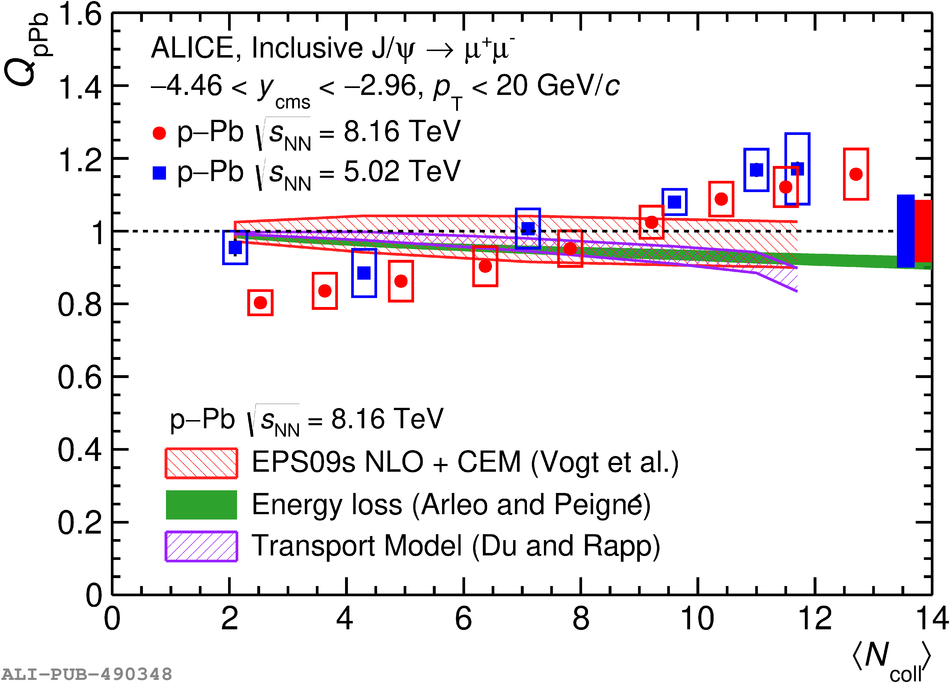# Figure 4

 Inclusive J/$\psi$ $Q_{\rm pPb}$ as a function of $\langle N_{\rm coll} \rangle$ at backward (left) and forward (right) rapidity in p--Pb collisions at $\sqrt{s_{\rm NN}} = 8.16$ TeV compared with the results at $\sqrt{s_{\rm NN}} = 5.02$ TeV  and theoretical models . The vertical error bars represent the statistical uncertainties and the boxes around the data points the uncorrelated systematic uncertainties. The boxes centered at $Q_{\rm pPb} = 1$ represent the systematic uncertainties correlated over centrality.